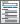»  Home »  Products »  Features »  Panel data

Panel/longitudinal data

Take full advantage of the extra information that panel data provide, while simultaneously handling the peculiarities of panel data. Study the time-invariant features within each panel, the relationships across panels, and how outcomes of interest change over time. Fit linear models or nonlinear models for binary, count, ordinal, censored, or survival outcomes with fixed-effects, random-effects, or population-averaged estimators. Fit dynamic models or models with endogeneity. And much more.

Random-effects regression for binary, ordinal, and count-dependent variables

*Robust standard errorsConditional fixed-effects regression for binary and count-dependent variables

Two-stage least-squares panel-data estimators• Between-2SLS estimator
• Within-2SLS estimator
• Balestra–Varadharajan–Krishnakumar G2SLS estimator
• Baltagi EC2SLS estimator
• All with balanced or exogenously balanced panels
• Robust and cluster–robust standard errors

Regressors correlated with individual-level effects• Hausman–Taylor instrumental-variables estimators
• Amemiya–MaCurdy instrumental-variables estimators
• Robust and cluster–robust standard errors

Panel-corrected standard errors (PCSE) for linear cross-sectional modelsSwamy’s random-coefficients regressionStochastic frontier models• Time-invariant model
• Time-varying decay model
• Battese–Coelli parameterization of time effects
• Estimates of technical efficiency and inefficiency

Specification tests

• Im–Pesaran–Shin
• Levin–Lin–Chu
• Breitung
• Fisher-type (combining p-values)
• Harris–Tzavalis

Summary statistics and tabulations

Panel-data line plots• Graphs by panel
• Overlaid panels

Linear dynamic panel-data estimators

• Weibull, exponential, lognormal, loglogistic, or gamma models
• Robust and cluster–robust standard errors
• Bayesian estimation

Population-averaged regression

Stationarity tests

• View and run all postestimation features for your command
• Automatically updated as estimation commands are run
• Automatically create indicators based on categorical variables
• Form interactions among discrete and continuous variables Updated
• Include polynomial terms
• Perform contrasts of categories/levels
Watch Introduction to Factor Variables in Stata tutorials

Marginal analysisWatch Introduction to margins in Stata tutorials
Watch Profile plots and interaction plots in Stata tutorials